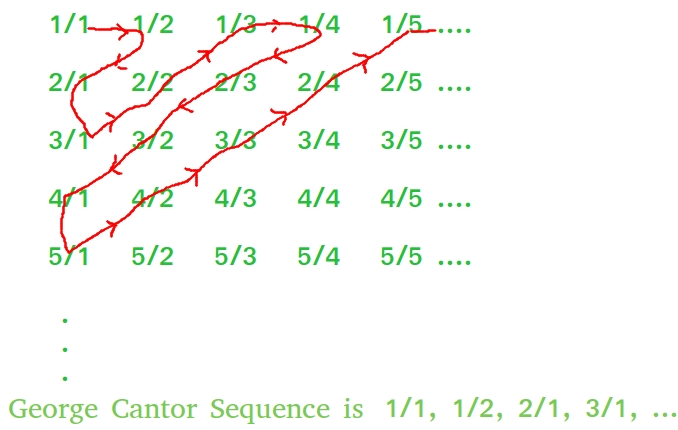Related Articles

# N-th term of George Cantor set of rational numbers

• Difficulty Level : Easy
• Last Updated : 07 Apr, 2021

George cantor named mathematician gave a proof that set of rational numbers is enumerable. We don’t have to proof it over here rather we have to determine the Nth term in the set of rational numbers.
Examples :

```Input : N = 8
Output : 2/3

Input : N = 15
Output : 1/5
See image for reference of counting.```

The set of rational numbers goes like the below image :Here the first term is 1/1, second term is 1/2, third term is 2/1, fourth term is 3/1, fifth term is 2/2, sixth term is 1/3 so on……

1. View this as a matrix(2-D array) with rows from 1 to n and columns from 1 to n.
2. rows/columns will give the rational numbers.
3. Observe the pattern from the above image it will be clear how to traverse through the matrix i.e diagonally from left to right upwards and then from right to left downwards till we reach the Nth position.
4. There are 4 regular repeating pattern
5. After each iteration move to next number to keep counting its position using counter k, then compare it with N

## C++

 `// C++ program to find N-th term in``// George Cantor set of rational numbers``#include ``using` `namespace` `std;` `void` `georgeCantor(``int` `n)``{    ``    ``int` `i = 1; ``// let i = numerator``    ``int` `j = 1; ``// let j = denominator``    ``int` `k = 1; ``// to keep the check of no. of terms``        ` `    ``// loop till k is not equal to n``    ``while` `(k < n)``    ``{``        ``j++ , k++;``        ` `        ``// check if k is already equal to N``        ``// then the first term is the required``        ``// rational number``        ``if` `(k == n)``            ``break``;``        ` `        ``// loop for traversing from right to left``        ``// downwards diagonally``        ``while` `(j > 1 && k < n) {``            ``i++, j--, k++;``        ``}``        ` `        ``if` `(k == n)``           ``break``;``        ` `        ``i++, k++;``        ` `        ``if` `(k == n)``           ``break``;``        ` `        ``// loop for traversing from left``        ``// to right upwards diagonally``        ``while` `(i > 1 && k < n) {``            ``i--, j++, k++;``        ``}       ``    ``}   ``    ``cout << ``"N-th term : "``<

## Java

 `// Java program to find N-th term in``// George Cantor set of rational number``import` `java.io.*;` `class` `GFG``{``    ``static` `void` `georgeCantor(``int` `n)``    ``{``        ``// let i = numerator``        ``int` `i = ``1``;``        ` `        ``// let j = denominator``        ``int` `j = ``1``;``        ` `        ``// to keep the check of no. of terms``        ``int` `k = ``1``;``            ` `        ``// loop till k is not equal to n``        ``while` `(k < n)``        ``{``            ``j++ ;``            ``k++;``            ` `            ``// check if k is already equal to N``            ``// then the first term is the required``            ``// rational number``            ``if` `(k == n)``                ``break``;``            ` `            ``// loop for traversing from right to left``            ``// downwards diagonally``            ``while` `(j > ``1` `&& k < n) {``                ``i++;``                ``j--;``                ``k++;``            ``}``            ` `            ``if` `(k == n)``            ``break``;``            ` `            ``i++;``            ``k++;``            ` `            ``if` `(k == n)``            ``break``;``            ` `            ``// loop for traversing from left``            ``// to right upwards diagonally``            ``while` `(i > ``1` `&& k < n) {``                ``i--;``                ``j++;``                ``k++;``            ``}``        ``}``        ``System.out.println(``"N-th term : "``+i +``"/"` `+j);``    ``}``    ` `    ``// Driver code``    ``public` `static` `void` `main (String[] args)``    ``{``        ``int` `n = ``15``;``        ``georgeCantor(n);``        ` `    ``}``}` `// This code is contributed by vt_m`

## Python3

 `# Python3 program to find N-th term in``# George Cantor set of rational numbers` `def` `georgeCantor(n):``    ` `    ``# let i = numerator``    ``i ``=` `1``    ` `    ``# let j = denominator``    ``j ``=` `1` `    ` `    ``# to keep the check of no. of terms``    ``k ``=` `1` `        ` `    ``# loop till k is not equal to n``    ``while` `k < n:``        ``j ``+``=` `1``        ``k ``+``=` `1``        ` `        ``# check if k is already equal to N``        ``# then the first term is the``        ``# required rational number``        ``if` `k ``=``=` `n:``            ``break``        ` `        ``# loop for traversing from right``        ``# to left downwards diagonally``        ``while` `j > ``1` `and` `k < n:``            ``i ``+``=` `1``            ``j ``-``=` `1``            ``k ``+``=` `1``        ` `        ``if` `k ``=``=` `n:``            ``break``        ` `        ``i ``+``=` `1``        ``k ``+``=` `1``        ` `        ``if` `k ``=``=` `n:``            ``break``        ` `        ``# loop for traversing from left``        ``# to right upwards diagonally``        ``while` `i > ``1` `and` `k < n:``            ``i ``-``=` `1``            ``j ``+``=` `1``            ``k ``+``=` `1``    ``print` `(``"N-th term : %d/%d"``%``(i, j))` `# Driver code``n ``=` `15``georgeCantor(n)` `# This code is contributed``# by Shreyanshi Arun`

## C#

 `// C# program to find N-th term in``// George Cantor set of rational number``using` `System;` `class` `GFG``{``    ``static` `void` `georgeCantor(``int` `n)``    ``{``        ``// let i = numerator``        ``int` `i = 1;``        ` `        ``// let j = denominator``        ``int` `j = 1;``        ` `        ``// to keep the check of no. of terms``        ``int` `k = 1;``            ` `        ``// loop till k is not equal to n``        ``while` `(k < n)``        ``{``            ``j++ ;``            ``k++;``            ` `            ``// check if k is already equal to N``            ``// then the first term is the required``            ``// rational number``            ``if` `(k == n)``                ``break``;``            ` `            ``// loop for traversing from right to left``            ``// downwards diagonally``            ``while` `(j > 1 && k < n)``            ``{``                ``i++;``                ``j--;``                ``k++;``            ``}``            ` `            ``if` `(k == n)``            ``break``;``            ` `            ``i++;``            ``k++;``            ` `            ``if` `(k == n)``            ``break``;``            ` `            ``// loop for traversing from left``            ``// to right upwards diagonally``            ``while` `(i > 1 && k < n)``            ``{``                ``i--;``                ``j++;``                ``k++;``            ``}``        ``}``        ``Console.WriteLine(``"N-th term : "``+i +``"/"` `+j);``    ``}``    ` `    ``// Driver code``    ``public` `static` `void` `Main ()``    ``{``        ``int` `n = 15;``        ``georgeCantor(n);``        ` `    ``}``}` `// This code is contributed by vt_m`

## PHP

 ` 1 && ``\$k` `< ``\$n``)``        ``{``            ``\$i``++; ``\$j``--; ``\$k``++;``        ``}``        ` `        ``if` `(``\$k` `== ``\$n``)``        ``break``;``        ` `        ``\$i``++; ``\$k``++;``        ` `        ``if` `(``\$k` `== ``\$n``)``        ``break``;``        ` `        ``// loop for traversing from left``        ``// to right upwards diagonally``        ``while` `(``\$i` `> 1 && ``\$k` `< ``\$n``)``        ``{``            ``\$i``--; ``\$j``++; ``\$k``++;``        ``}``    ``}``    ``echo` `"N-th term : "``, ``\$i``, ``"/"``, ``\$j``;``}` `// Driver Code``\$n` `= 15;``georgeCantor(``\$n``);` `// This code is contributed by ajit``?>`

## Javascript

 ``

Output :

`N-th term : 1/5`

Attention reader! Don’t stop learning now. Get hold of all the important mathematical concepts for competitive programming with the Essential Maths for CP Course at a student-friendly price. To complete your preparation from learning a language to DS Algo and many more,  please refer Complete Interview Preparation Course.

My Personal Notes arrow_drop_up